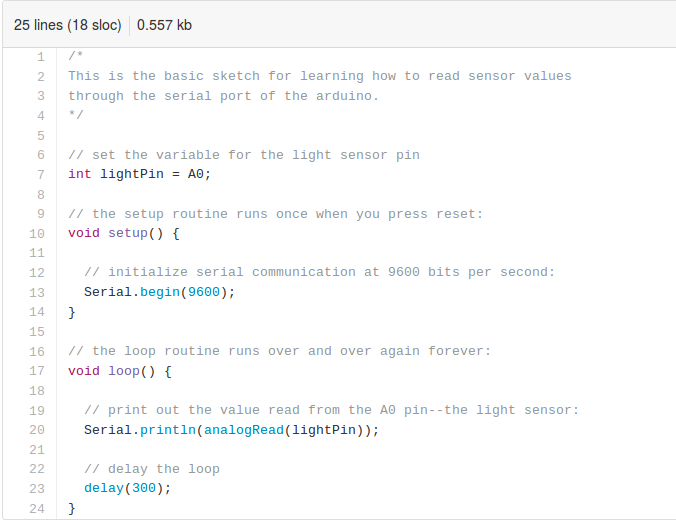It looks like you're using Internet Explorer 11 or older. This website works best with modern browsers such as the latest versions of Chrome, Firefox, Safari, and Edge. If you continue with this browser, you may see unexpected results.

# Arduinos: Photocell Software

Physical Computing, the Internet of Things, Sensors and Servos

## Introduction

The software portion of this training is a very basic introduction to programming an Arduino microcontroller. Arduinos use a variation of the Wiring programming language, and Wiring is based on the Processing programming environment. Today's training will explain how to construct a basic Arduino script. There are two basic components of an Arduino script: void setup() and, (2) void loop().

In the basic below, used for reading values from a photocell through the serial port of the Arduino, the two basic components listed above are included:• The void setup() method is used to setup the connection between the Arduino board and the code that controls it. In the case of the code above, the Serial.begin(9600) function on line 13 in the code above tells the Arduino to communicate over the serial port at a data rate (baud) of 9600 bits per second.
• The void loop() method is where the actual activity of the program affects the Aruino, or various components connected to the Arduino, in some way. In the case of the code above, it tells the Arduino board with the analogRead() function to read the lightPin variable.
• At the very top of the script, line 7 reads: int lightPin = A0; and this sets the lightPin variable equal to the A0 pin on the Arduino board. Therefore, Serial.println() prints to the serial monitor the value that it reads from the A0 pin, and this is what is displayed on line 20 of the code above.
• Finally, the last line of the code delays the loop by 300 miliseconds with the delay() function. Without this, the values printed to the serial monitor change too quickly to be easily monitored during prototyping.

The code above and a second script below form the basis for today's training. Please go to the GitHub page below and download the ZIP file containing the two scripts. See screen shot below link for additional help.

https://github.com/aaronbev79/Arduino_TrainingAfter downloading the files, open the Arduino IDE and open the serial_monitor script in the IDE. Playing with this script will provide an introduction to uploading code to the Arduino, and how to read sensor values from the serial monitor.  The hands-on portion of this portion of the training will begin now.

## Photocell Code

This part of the training focuses on the code that controls the LED based on reading values from the Photocell (light sensor). This second section assumes that participants in the training have adequately constructed a breadboard layout as presented in the "hardware" section of this training, and it also assumes that participants have downloaded the ZIP file mentioned in the first component of the "software" section of this training.

Here is the code that will be uploaded and modified during this component of the training:• There are two new functions included in this code that are new: pinMode() and digitalWrite(). The pinMode() function appears in the setup loop() method because this function tells the Arduino board how to treat the actual physical pins on the board. On line 19 in the code above, pinMode() function sets the photoPin variable to INPUT. As we see at the top of the code on line 10, the photoPin variable is linked to the A0 pin on the Arduino board. Therefore, line 19 tells the Arduino that the A0 pin on the Arduino board will be reading some sort of INPUT value.
• The second part of the setup loop() is on line 23 in the code above. This line in the code sets the variable ledPin to OUTPUT. This tells the Arduino board that this pin will be sending some sort of output to this pin. Just like line 19, the ledPin is set to OUTPUT by using the pinMode() function.
• The void loop() method in the script above uses an if/else statement to determine how to control the Arduino. The first part of the "software" training addressed the analogRead() function, but there is a new function introduced in this part of the script: digitalWrite(). Since we told the Arduino that the ledPin variable is set to OUTPUT, the digitalWrite() function provides a basic "off" or "on" OUTPUT to the ledPin. In Arduino programming, "off" is usually designated by the LOW argument, and "on" is designated by the HIGH argument.
• The key component of the if/else statement in this code is the threshold variable. In the first section of the "software" component of this tutorial, we used the serial monitor to determine the dark/light threshold for each of the photocell sensors used in this tutorial. On line 32 of the code above, the value of the threshold variable is compared to the reading from the photoPin variable. If the photoPin variable is greater than the threshold variable, then the ledPin is set to "off" or LOW. However, if the photoPin variable is less than the threshold variable, then the ledPin is set to "on" or HIGH.

Now begins the second hands-on component for the "software" section of this tutorial.